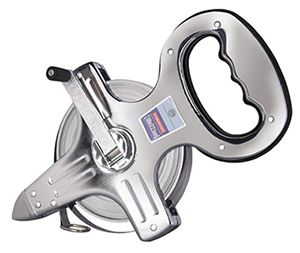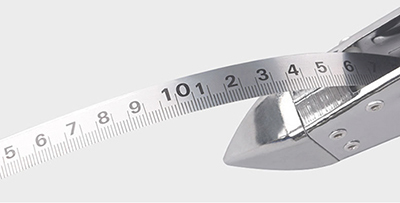Search
All Categories

# How to Read a Steel Tape Measure?

The steel tape measure is measured in centimeters. The red “10” means 10 centimeters, and the cells in centimeters mean millimeters. Steel tapes can be divided into self-rolling tapes, braking tapes, and rolling tapes. The numbers on the metal tape measure are divided into two rows, the unit of one row is cm, and the unit of the other row is inches. 1cm≈0.3937 inches, 1 inch≈2.54cm. Therefore, when the difference between the two numbers is small, the unit uses centimeters, and when the difference is large, the unit uses inches. The font of numbers in centimeters is also smaller than that of inches.When measuring, it is necessary to align the zero scale of the steel tape with the starting point of the measurement, and apply appropriate tension, and then directly read the scale of the steel tape corresponding to the end point of the measurement.

In some parts where steel tape measure cannot be used directly, other tape measure or square ruler can be used. At this time, only need to align the zero scale with the measuring point, and the ruler body should be consistent with the measuring direction. It is necessary to use a steel tape measure to measure the distance to a certain full scale on a steel rule or square, and the excess length is measured by the reading method.

Several errors in steel tape measurement
The more accurate steel tape must be verified when it leaves the factory and after a period of use, and the temperature, tensile force and ruler length shall be indicated at the time of verification. And the 30m open reel steel tape measure for sale on Tool.com has been tested before leaving the factory, you can buy it with confidence. The length marked on the ruler is the nominal length, and the difference between it and the actual length is called the ruler length correction Δl. The length of the ruler will change when the ruler is subjected to different pulling forces. In order to avoid this change, it is required to use the steel tape in accordance with the tensile force marked on the ruler. The length of the steel tape will change at different temperatures, so a function with the temperature t as a variable must be used to express the length. This is the ruler length equation. Its general form is:
lt=l+Δl+α·l(t-t0)In the formula, it is the practical length of the steep tape at the temperature of t;
I is the nominal length of the steel tape;
Δl is the length correction, which means the difference between the practical length and nominal length at the temperature of t0;
α: Thermal expansion coefficient of steel tape;
t0: The temperature of the steel tape at the time of verification;
t: The temperature of the steel tape in use.

• Error of temperature change
The thermal expansion coefficient of a general steel tape measure is α=1.25x10-5, the temperature difference per meter per degree Celsius changes only one eighty thousandth. However, the same steel tape will still produce a large length change in an environment with a large temperature difference, which will affect the measurement results. The error of temperature change has been considered in the ruler length equation.
• Tension error
The pulling force will affect the length of the steel tape. When measuring, if the spring scale is not used to measure the tension, errors will occur. According to Hooke's law, a 30-meter ruler will produce a length error of ±1.8 mm when the error of ±5 kg pull force.
• Error of not leveled steel tape
When measuring the horizontal distance, the steel tape should be kept as level as possible, otherwise the distance increase error will occur. For a ruler length of 30 meters, when the height difference between the two ends of the ruler reaches 0.4 meters, an error of about 2.6 mm will occur. The relative error is 1/11200.
Write a comment Close